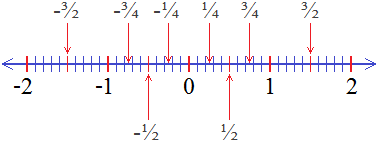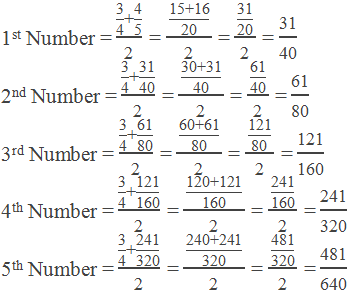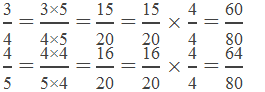# Rational Numbers## What is a Rational Number?

The definition of a rational number is “A number which can be expressed in the form of p/q or fraction where p and q are integers and q ≠ 0 is a Rational Number.

********************

10 Math Problems officially announces the release of Quick Math Solver, an Android App on the Google Play Store for students around the world.

********************### Examples of Rational Numbers

All the integers and decimal numberes can be expressed in the form of p/q or fraction of two integers. For example 0 = 0/1, 1 = 2/2, -2 = -4/2, 0.5 = ½, 1.25 = 5/4, 0.3333… = 1/3 etc. Therefore, 0, 1, -2, 0.5, 1.25, 0.3333… etc. are some examples of rational numbers

Rational numbers include all the integers on a number line along with the fractions between integers. Look at the number line given below.The set of rational numbers is denoted by Q.

Q = {… -1, …, -¾, …, -½, …, -¼, …, 0, …, ¼, …, ½, …, ¾, …, 1, …}

Note:

-      There are infinitely many rational numbers between any two integers.

-      There are infinitely many rational numbers between any two rational numbers.

### How to find rational numbers between two numbers?

We can find rational numbers between any two numbers by the following two methods:

1.     By Mean Method

2.     By Fraction Method

#### By Mean Method

In this method, we find the middle value i.e. mean of the given two numbers by dividing their sum by 2. Then again, we find the mean of the first and the middle number by dividing their sum by 2. And, by continuing this procedure we can find any number of rational numbers between them.

Look at the following examples of finding rational numbers by mean method.

Example 1: Find 6 rational numbers between 3 and 4.

Solution: Here,

Six rational numbers between 3 and 4 by mean method are as follows:

1st Number = (3+4)/2 = 7/2 = 3.5

2nd Number = (3+3.5)/2 = 6.5/2 = 3.25

3rd Number = (3+3.25)/2 = 6.25/2 = 3.125

4th Number = (3+3.125)/2 = 6.125/2 = 3.0625

5th Number = (3+3.0625)/2 = 6.0625/2 = 3.03125

6th Number = (3+3.03125)/2 = 6.03125/2 = 3.015625

3.5, 3.25, 3.125, 3.0625, 3.03125 and 3.015625 are 6 rational numbers between 3 and 4.

Example 2: Find five rational numbers between 3/4 and 4/5.

Solution: Here,

Five rational numbers between 3/4 and 4/5 by the mean method are as follows:∴ Five rational numbers between 3/4 and 4/5 are:#### By Fraction Method

In this method, we convert the given numbers into the fractions of equal denominators, then multiply the numerator and denominator by n+1 to both the fractions to obtain n rational numbers between them.

Look at the following examples of finding rational numbers by fraction method.

Example 3: Find five rational numbers between 1 and 2.

Solution: Here,

We need 5 rational numbers between 1 and 2. So, we have to multiply 6/6 to both the numbers to obtain 5 rational numbers between them.In between 6 and 12, there are 7, 8, 9, 10, and 11. Therefore, five rational numbers between 1 and 2 are:Example 4: Find 3 rational numbers between 3/4 and 4/5.

Solution: Here,

We can convert 3/4 and 4/5 into the rational number of common denominator 20 by multiplying first by 5 and second by 4. Then, we have to multiply 4/4 to both the rational numbers to obtain 3 more rational numbers between them as follows.In between 60 and 64, there are 61, 62, and 63. Therefore, 3 rational numbers between ¾ and 4/5 are:### Properties of Rational Numbers

Following are the properties of Rational Numbers:

1.    Rational Numbers are closed under the operation of Addition, Subtraction, Multiplication and Division.

i.e. if x, y Q, then x+y Q, x–y Q, x×y Q and x÷y Q.

2.    Rational Numbers are Commutative.

i.e. if x, y Q, x+y = y+x and x×y = y×x

3.    Rational Numbers are Associative.

i.e. if x, y, z Q, (x+y)+z = x+(y+z)

4.    Rational Numbers are Distributive.

i.e. if x, y, z Q, x×(y+z) = x×y + x×z

5.    There is an Additive Identity 0, such that x+0=0+x=x.

6.    There is a Multiplicative Identity 1, such that x×1=1×x=x.

7.    There exist an Additive Inverse for every Rational Number.

i.e. if x Q there exist -x Q such that x+(-x)=(-x)+x=0 (Additive Identity)

8.    There exist a Multiplicative Inverse for every Rational Number.

i.e. if x Q there exist 1/x Q such that x×1/x=1/x×x=1 (Multiplicative Identity)

### Rational Numbers and Decimals

When we convert a rational number into the decimal form, the number so obtained may be terminating or non terminating decimal. In the case of non-terminating decimal, a digit or a block of digits after the decimal point gets repeated after certain intervals. Such decimals are termed non-terminating recurring decimals. For example:

1/3 = 0.3333333…

5/11 = 0.454545…

But others decimals that get terminated are called terminating decimals. For example:

1/4 = 0.25

2/5 = 0.4

### Questions and Answers on Rational Numbers

#### Is 0 a rational number?

Yes, 0 is a rational number because it can be expressed in the form of p/q like 0/1, 0/2, 0/3, etc.

#### Is 1 a rational number?

Yes, 1 is a rational number because it can be expressed in the form of p/q like 1/1, 2/2, 3/3, etc.

#### Is 2 a rational number?

Yes, 2 is a rational number because it can be expressed in the form of p/q like 2/1, 4/2, 6/3, etc.

#### Is 3 a rational number?

Yes, 3 is a rational number because it can be expressed in the form of p/q like 3/1, 6/2, 9/3, etc.

#### Is 4 a rational number?

Yes, 4 is a rational number because it can be expressed in the form of p/q like 4/1, 8/2, 12/3, etc.

#### Is 5 a rational number?

Yes, 5 is a rational number because it can be expressed in the form of p/q like 5/1, 10/2, 15/3, etc.

#### Is pi(π) a rational number?

No, pi(π) is not a rational number. 22/7 is not the exact value of pi. It is just an approximation. The value of pi(π) is non-terminating and non-recurring decimal 3.14159265358979323846… which is an irrational number.

If you have any questions or problems regarding the Rational Numbers, you can ask here, in the comment section below.